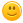+ Нов въпросStanimirPavlov 4 Точки

## 5. Extract an Non-decreasing Subsequence from an Array - Javascript

Здравейте,

На задача 5. Extract an Non-decreasing Subsequence from an Array не мога да извадя повече от 60/100. Условие:

Write a JS function that extracts only those numbers that form a non-decreasing subsequence. In other words, you start from the first element and continue to the end of the given array of numbers. Any number which is LESS THAN the current biggest one is ignored, alternatively if it’s equal or higher than the current biggest one you set it as the current biggest one and you continue to the next number.

The input comes as array of strings. Each element will represent a number.

The output is the processed array after the filtration, which should be a non-decreasing subsequence. Each element should be printed on a new line.

Предварително благодаря

Тагове:
1fbinnzhivko 1586 Точки

https://pastebin.com/H9Z5FdKS

Сега този код дава 80/100 като съм карал курса е давал 100/100.
Или някои тест е счупен или са променяли пак нещо в Judje.

1StanimirPavlov 4 Точки

Благодаря!

0YavorSpassov+deleted! 133 Точки

Моето дава 100%, както и това на fbinnzhivko. Явно има значение по кое време събмитваш - сутрин рано или късно вечер.``````function main(lines) {
lines=lines.map(Number);
let result=[];
let biggest = lines;
for (let i = 0; i < lines.length; i++) {
if(lines[i]>=biggest){
result.push(lines[i]);
biggest=lines[i];
}
}
console.log(result.join('\n'));
}``````

0StanimirPavlov 4 Точки

Много благодаря!

0krum_43 743 Точки

Може да завъртиш итератора в цикъла и от 1 .

0lorishte 2 Точки

Малко късно, но ето и моето решение. Даваше ми грешка на един от тестовете, докато разбера, че следващото число може да е по-голямо или равно на предишното.

Някой успя ли да реши задачата с arr.filter(), както е дадено в подсказката на условията?

0JivkoJelev 235 Точки

Да и на мен ще ми е много интересно да вида решение с филтър.Доста по-кратък и четим ще е кода.Лошото е че така и не го измислих.

0RoYaL Trainer 6848 Точки

Ще е по-кратък, но няма да е четим. Дайте линк към задачата в Judge. Иначе би трябвало да е нещо такова (по условието от първия пост):

``(arr, biggestNumber = -Infinity) => arr.filter(e => ([ret, biggestNumber] = [parseInt(e) >= biggestNumber, Math.max(biggestNumber, e)])).join('\n');``

3
01/06/2017 16:28:04lorishte 2 Точки

Явно става, но наистина не е добре четим.

Ето линк към Judge: https://judge.softuni.bg/Contests/Compete/Index/313#4

Даде 100/100 :)

0
02/06/2017 07:34:27Pittyr 3 Точки

Макар и поста да е от 2017 задачата все още я има в More Exercise, затова реших да споделя няколко решения, които могат да помогнат на някой.

1. Решение с filter

``````function solve(array) {
let max = Number.MIN_SAFE_INTEGER;
let arr = array.filter(num => {
if (num > max) {
max = num;
return true;
} else {
return false;
}
});

console.log(arr.join(" "));
};``````

2. Решение с filter и допълнителна фунция

``````function solve(array) {
let max = Number.MIN_SAFE_INTEGER;
let arr = array.filter(filterMaxNum);

function filterMaxNum(num) {
if (num >= max) {
max = num;
return true;
}
return false;
}

console.log(arr.join(" "));
};``````

3. Решение с for и push

``````function solve(array) {
let newArr = [];
let max = Number.MIN_SAFE_INTEGER;

for (let i = 0; i < array.length; i++) {
if (array[i] >= max) {
max = array[i];
newArr.push(array[i]);
}
}

console.log(newArr.join(" "));
};``````

1MariqnK1 52 Точки

Колеги гърми ми 4ти тест с грешен отговор. В описанието е подсказано, че може с reduce, но не го разбирам. Моето решение изглежда адекватно и self-explanatory, но задачата явно е направена за reduce.

Една подсказка, ако може как ще стане с reduce? Не е нужно да поствате готово решение. Благодаря!

https://judge.softuni.bg/Contests/Practice/Index/1798#4

https://pastebin.com/paB5QPPG

0AtillaOmer 13 Точки
``````function solve(array) {
let result = array.reduce((arr, current) => {
if (arr.length) {
if (current >= arr[arr.length - 1]) {
arr.push(current);
}
} else {
arr.push(current);
}
return arr;
}, []);
return result;
}``````

0
25/09/2021 11:42:55djambazki 3 Точки

Здравейте, колеги, ето решение с метода reduce().

``````function solve(arr){
arr.reduce((acc, val) => {
if(val >= acc){
console.log(val);
acc = val;
}

return acc;
}, Number.MIN_SAFE_INTEGER)
}``````

или

``````function solve(args){
let max = Number.MIN_SAFE_INTEGER;

let output = args.reduce((acc, val) => {
if(val >= max){
max = val;
acc.push(max);
}

return acc;
}, [])

output.forEach(x => console.log(x));
}``````

0
29/09/2020 15:51:02cecosvidovski 58 Точки

Гърми с 80/100 на 4ти тест.

0AtillaOmer 13 Точки
``````function solve(array) {
let result = array.reduce((arr, current) => {
if (arr.length) {
if (current >= arr[arr.length - 1]) {
arr.push(current);
}
} else {
arr.push(current);
}
return arr;
}, []);
return result;
}``````

0NikolaMihaylov06 2 Точки

function nonDecreasing(arr) {

let biggest = Number.MIN_SAFE_INTEGER;

let resultArr = [];

arr.filter(check);

function check(a) {

if (a >= biggest) {

biggest = a;

resultArr.push(a);

}

}

console.log(resultArr.join(" "));

}

1
Можем ли да използваме бисквитки?
Ние използваме бисквитки и подобни технологии, за да предоставим нашите услуги. Можете да се съгласите с всички или част от тях.
Назад
Функционални
Използваме бисквитки и подобни технологии, за да предоставим нашите услуги. Използваме „сесийни“ бисквитки, за да Ви идентифицираме временно. Те се пазят само по време на активната употреба на услугите ни. След излизане от приложението, затваряне на браузъра или мобилното устройство, данните се трият. Използваме бисквитки, за да предоставим опцията „Запомни Ме“, която Ви позволява да използвате нашите услуги без да предоставяте потребителско име и парола. Допълнително е възможно да използваме бисквитки за да съхраняваме различни малки настройки, като избор на езика, позиции на менюта и персонализирано съдържание. Използваме бисквитки и за измерване на маркетинговите ни усилия.
Рекламни
Използваме бисквитки, за да измерваме маркетинг ефективността ни, броене на посещения, както и за проследяването дали дадено електронно писмо е било отворено.